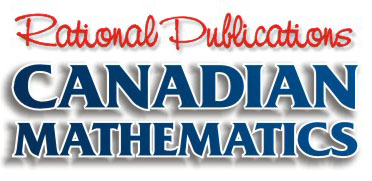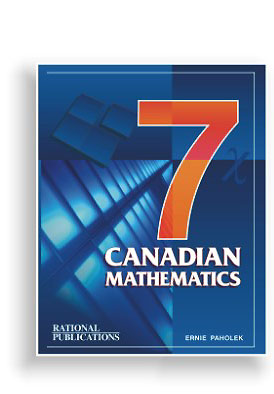The following summary contains a complete listing of the chapters, along with a sample question from each chapter.

Should you have further questions about the text book, please contact us.

If you wish to order Canadian Mathematics 7 or any of the other Rational Publications text books, please do so by clicking on the order form.

 CHAPTER 1 - WHOLE NUMBERS 1.1 Place Value of Whole Numbers 1.2 Expanded & Standard Form 1.3 Graphing Whole Numbers 1.4 Rounding & Estimation 1.5 Addition of Whole Numbers 1.6 Subtraction of Whole Numbers 1.7 Multiplication of Whole Numbers 1.8 Division of Whole Numbers 1.9 Properties of Whole Numbers 1.10 Review CHAPTER 2 - NUMBER EXPRESSIONS 2.1 Order of Operations (BEDMAS) 2.2 Substitution (Single Symbol) 2.3 Substitution (Double Symbols) 2.4 Algebraic Substitution 2.5 Sequences & Functions 2.6 Graphing Algebraic Functions 2.7 Review CHAPTER 3 - INTEGERS (I) 3.1 Positive & Negative Integers 3.2 Addition of Integers 3.3 Subtaction of Integers 3.4 Addition & Subtraction 3.5 Multiplication of Integers 3.6 Division of Integers 3.7 Multiplication & Division 3.8 Integers Review CHAPTER 4 - EQUATIONS (W) 4.1 Solving Type I Equations 4.2 Solving Type II Equations 4.3 Solving Type III Equations 4.4 Solving Type IV Equations 4.5 Solving Ratio Type Equations 4.6 Review CHAPTER 5 - TRANSLATION 5.1 Mathematics <—> English 5.2 Solving Number Problems 5.3 Solving Various Problems 5.4 Review CHAPTER 6 - FACTORS & MULTIPLES 6.1 Rules for Divisibility 6.2 Factors 6.3 Multiples 6.4 Prime & Composite Numbers 6.5 Relatively Prime Number 6.6 Prime Factorization 6.7 Greatest Common Factor (GCF) 6.8 Lowest Common Multiple (LCM) 6.9 Review CHAPTER 7 - FRACTIONS 7.1 Meaning of Fractions 7.2 Fractions on a Number Line 7.3 Equivalent Fractions 7.4 Basic Fractions 7.5 Improper & Mixed Fractions 7.6 Comparing & Order of Fractions 7.7 Addition of Fractions 7.8 Subtraction of Fractions 7.9 Adding & Subt. Mixed Fractions 7.10 Multiplication of Fractions 7.11 Multiplying Mixed Fractions 7.12 Division of Fractions 7.13 Dividing Mixed Fractions 7.14 Order of Operations - Fractions 7.15 Review CHAPTER 8 - DECIMALS 8.1 Expanded Form For Decimals 8.2 Converting Decimals —> Fractions 8.3 Converting Fractions —> Decimals 8.4 Order of Decimals 8.5 Graphing Decimals 8.6 Rounding Off Decimals 8.7 Adding and Subt. Decimals 8.8 Multiplication of Decimals 8.9 Division With Decimals 8.10 Order of Operations - Decimals 8.11 Review CHAPTER 9 - EQUATIONS (RATIONALS) 9.1 Solving Type I Equations 9.2 Solving Type II Equations 9.3 Solving Type III & IV Equations 9.4 Solving Ratio Equations 9.5 Review CHAPTER 10 - RATE, RATIO & PERCENT 10.1 Ratios 10.2 Equivalent Ratios 10.3 Fractions <—> Decimals <—> Percent 10.4 Ratio, Proportion & Rate 10.5 Percent Problems 10.6 Discount, Sale & Regular Price 10.7 Commission 10.8 Sales Tax 10.9 Percent Equations 10.10 Review CHAPTER 11 - EXPONENTS 11.1 Bases, Exponents & Powers 11.2 Powers of Ten 11.3 Order of Operations 11.4 Review CHAPTER 12 - GEOMETRY 12.1 Plane Geometry 12.2 Angles & Measurement 12.3 Polygons 12.4 Triangles 12.5 Quadrilaterals 12.6 Circles CHAPTER 13 - MOTION GEOMETRY 13.1 Tracing to Prove Congruency 13.2 The Slide 13.3 The Reflection 13.4 The Turn 13.5 Similar Figures 13.6 Review CHAPTER 14 - MEASUREMENT 14.1 The Metric System (Length) 14.2 Linear Conversions 14.3 Perimeter of Polygons 14.4 Perimeter Formulas 14.5 Area of Polygons 14.6 Area Formulas 14.7 Circles 14.8 Volume of Solids 14.9 Volume of Rectangular Prisms 14.10 Capacity & Mass 14.11 World Time Zones 14.12 Review CHAPTER 15 - DATA MANAGEMENT 15.1 Collecting & Organizing Data 15.2 Working With Data 15.3 Stem and Leaf Plots 15.4 The Pictograph 15.5 The Bar Graph 15.6 The Broken Line Graph 15.7 The Circle Graph 15.8 Probability 15.9 Review

Home   |   Math 8   |   Math 9    |   Order Form   |   Contact Us